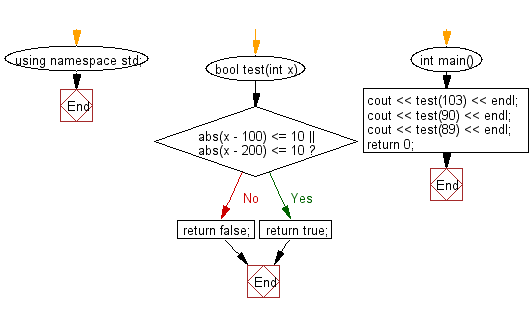﻿ C++ : Check a integer is within 10 of 100 or 200# C++ Exercises: Check a given integer and return true if it is within 10 of 100 or 200

## C++ Basic Algorithm: Exercise-4 with Solution

Write a C++ program to check a given integer and return true if it is within 10 of 100 or 200.

Sample Solution:

C++ Code :

``````#include <iostream>

using namespace std;

bool test(int x)
{
if(abs(x - 100) <= 10 || abs(x - 200) <= 10)
return true;
return false;
}

int main()
{
cout << test(103) << endl;
cout << test(90) << endl;
cout << test(89) << endl;
return 0;
}
``````

Sample Output:

```1
1
0
```

Flowchart:C++ Code Editor:

Contribute your code and comments through Disqus.

What is the difficulty level of this exercise?

﻿